• Slides: 52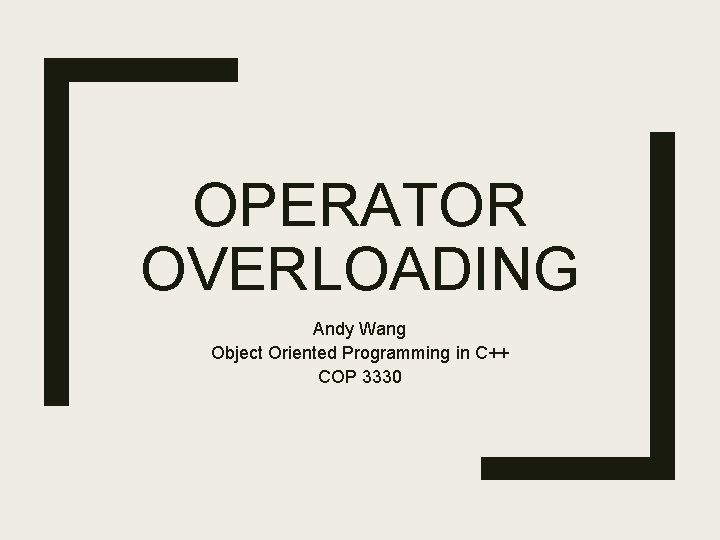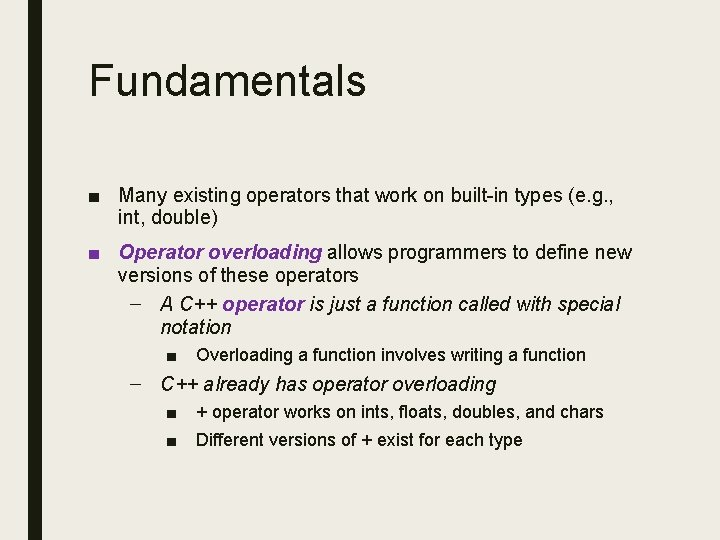Fundamentals ■ Many existing operators that work on built-in types (e. g. , int, double) ■ Operator overloading allows programmers to define new versions of these operators – A C++ operator is just a function called with special notation ■ Overloading a function involves writing a function – C++ already has operator overloading ■ ■ + operator works on ints, floats, doubles, and chars Different versions of + exist for each type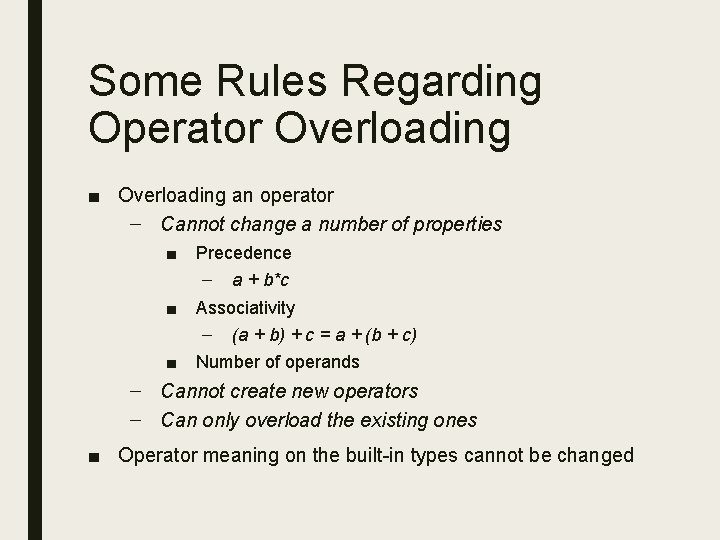Some Rules Regarding Operator Overloading ■ Overloading an operator – Cannot change a number of properties ■ ■ ■ Precedence – a + b*c Associativity – (a + b) + c = a + (b + c) Number of operands – Cannot create new operators – Can only overload the existing ones ■ Operator meaning on the built-in types cannot be changed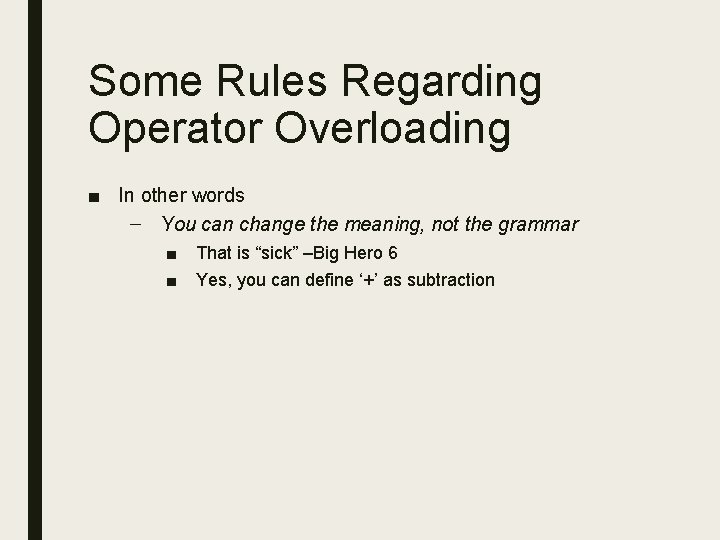Some Rules Regarding Operator Overloading ■ In other words – You can change the meaning, not the grammar ■ ■ That is “sick” –Big Hero 6 Yes, you can define ‘+’ as subtraction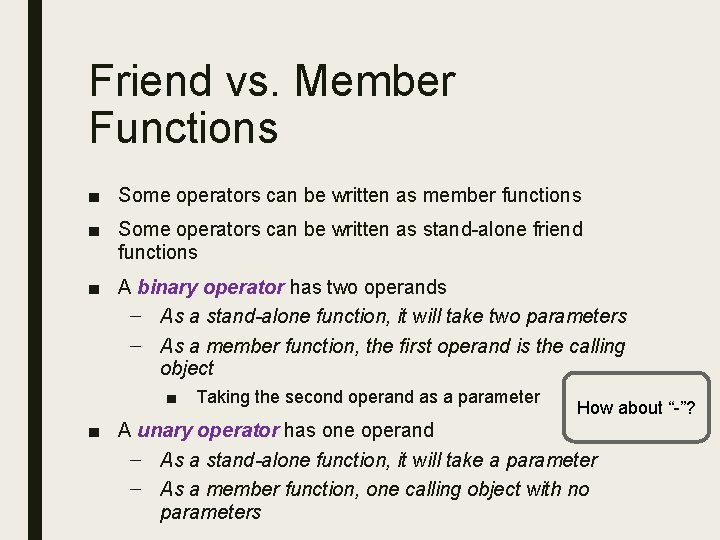Friend vs. Member Functions ■ Some operators can be written as member functions ■ Some operators can be written as stand-alone friend functions ■ A binary operator has two operands – As a stand-alone function, it will take two parameters – As a member function, the first operand is the calling object ■ Taking the second operand as a parameter How about “-”? ■ A unary operator has one operand – As a stand-alone function, it will take a parameter – As a member function, one calling object with no parameters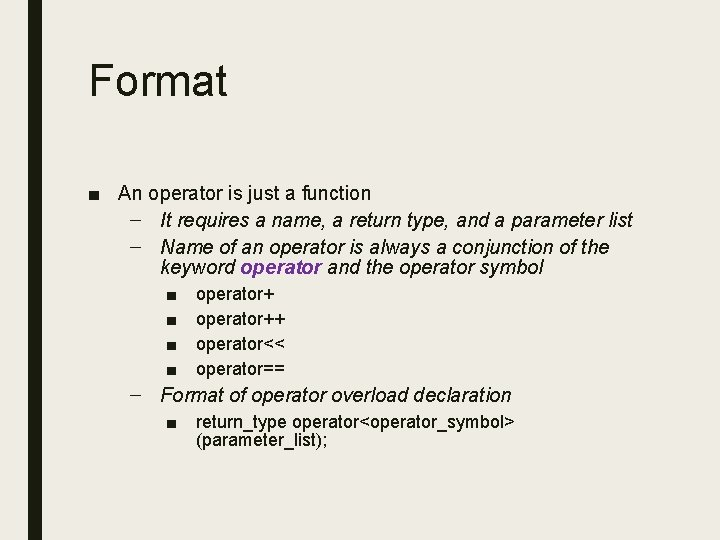Format ■ An operator is just a function – It requires a name, a return type, and a parameter list – Name of an operator is always a conjunction of the keyword operator and the operator symbol ■ ■ operator++ operator<< operator== – Format of operator overload declaration ■ return_type operator<operator_symbol> (parameter_list);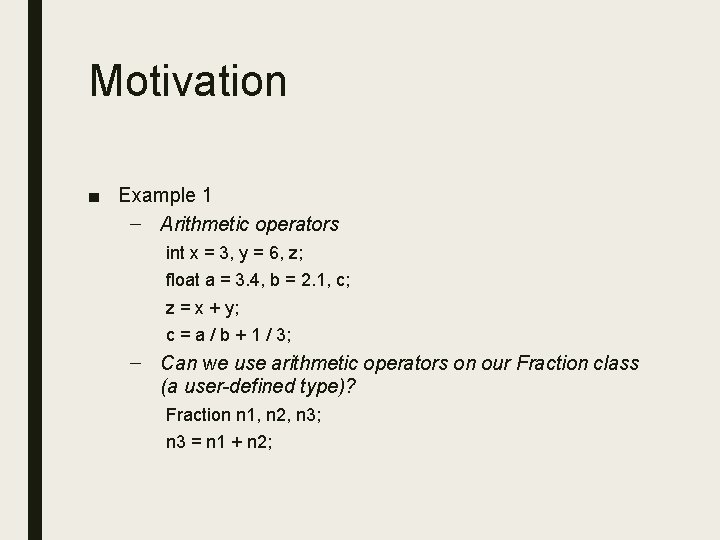Motivation ■ Example 1 – Arithmetic operators int x = 3, y = 6, z; float a = 3. 4, b = 2. 1, c; z = x + y; c = a / b + 1 / 3; – Can we use arithmetic operators on our Fraction class (a user-defined type)? Fraction n 1, n 2, n 3; n 3 = n 1 + n 2;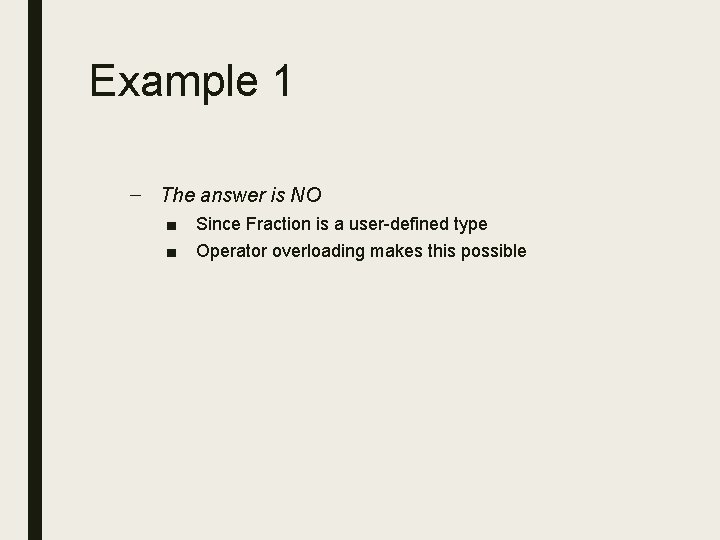Example 1 – The answer is NO ■ ■ Since Fraction is a user-defined type Operator overloading makes this possible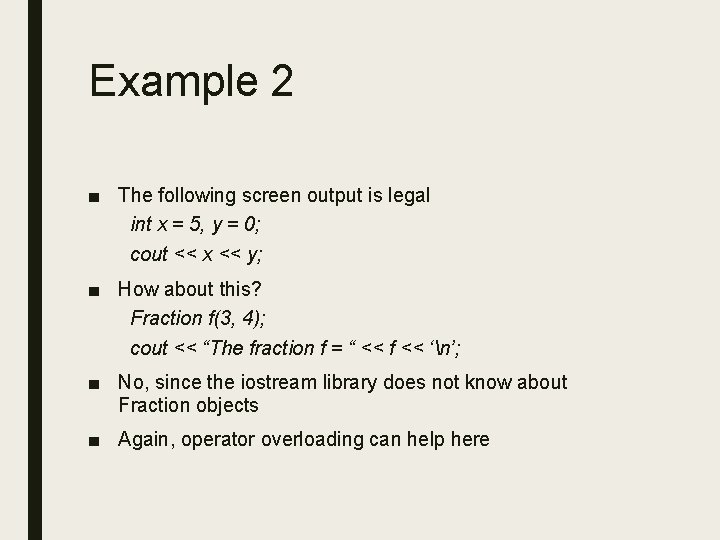Example 2 ■ The following screen output is legal int x = 5, y = 0; cout << x << y; ■ How about this? Fraction f(3, 4); cout << “The fraction f = “ << f << ‘n’; ■ No, since the iostream library does not know about Fraction objects ■ Again, operator overloading can help hereOverloading the Arithmetic Operators ■ Can be overloaded either as stand-alone functions or as member functions ■ Originally, we have the following friend Fraction Add(Fraction f 1, Fraction f 2); – To add, perform the following Fraction n 1, n 2, n 3; n 3 = Add(n 1, n 2);The + Operator ■ With operator overloading, we can have the following friend Fraction operator+(Fraction f 1, Fraction f 2); ■ Or the following friend Fraction operator+(const Fraction &f 1, const Fraction &f 2); ■ Thus, we can invoke the function this way n 3 = operator+(n 1, n 2); Note: const is only allowed for member functions ■ Or, we can use the infix notation n 3 = n 1 + n 2; // cascading also works (n 1 + n 2 + n 3 + n 4…)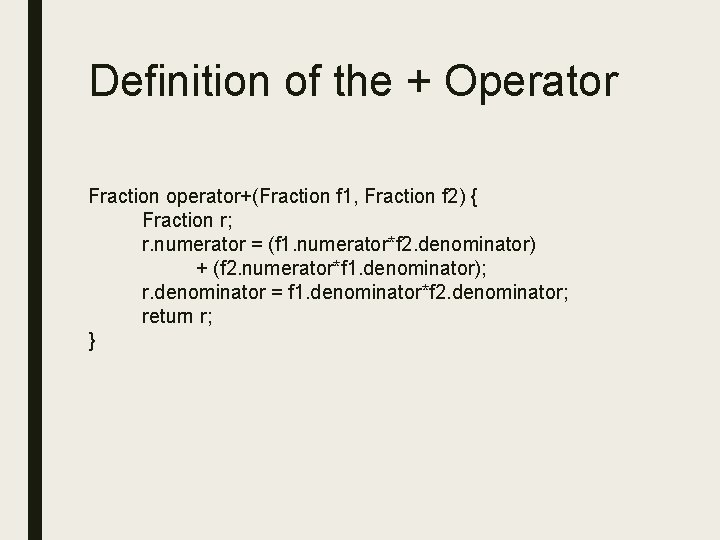Definition of the + Operator Fraction operator+(Fraction f 1, Fraction f 2) { Fraction r; r. numerator = (f 1. numerator*f 2. denominator) + (f 2. numerator*f 1. denominator); r. denominator = f 1. denominator*f 2. denominator; return r; }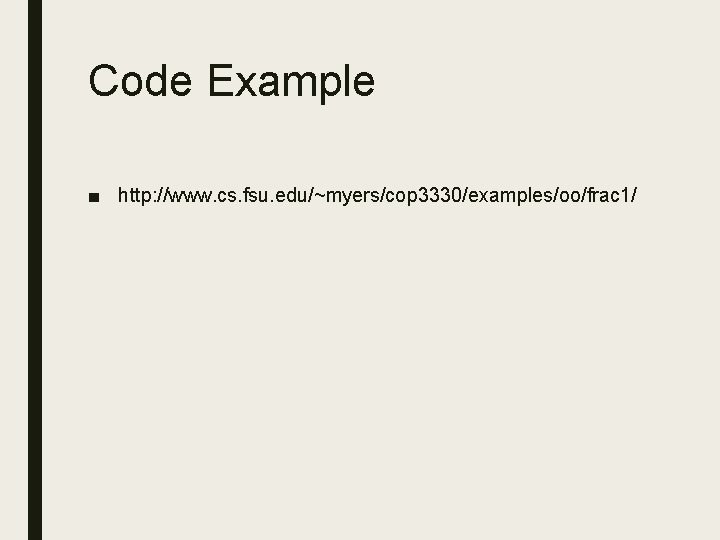Code Example ■ http: //www. cs. fsu. edu/~myers/cop 3330/examples/oo/frac 1/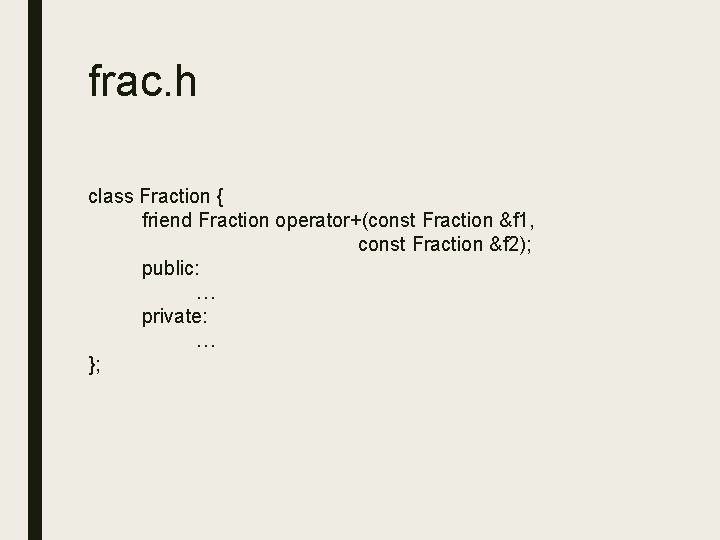frac. h class Fraction { friend Fraction operator+(const Fraction &f 1, const Fraction &f 2); public: … private: … };frac. cpp … Fraction operator+(const Fraction &f 1, const Fraction &f 2) { Fraction r; r. numerator = (f 1. numerator*f 2. denominator) + (f 2. numerator*f 1. denominator); r. denominator = f 1. denominator*f 2. denominator; return r; } …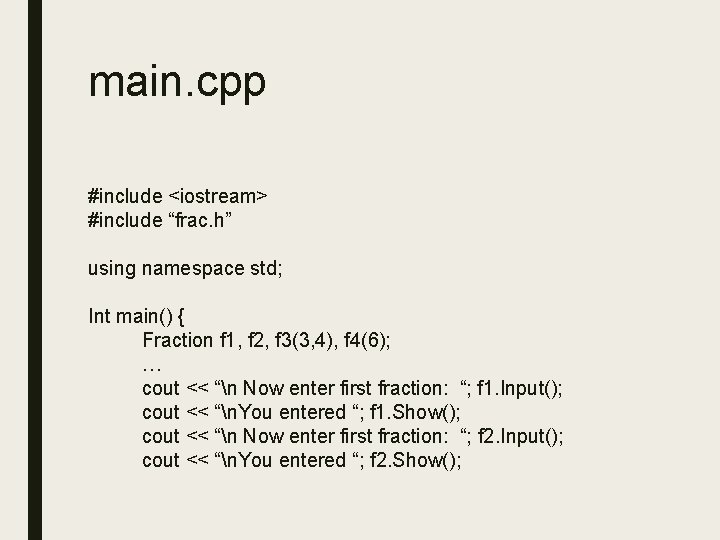main. cpp #include <iostream> #include “frac. h” using namespace std; Int main() { Fraction f 1, f 2, f 3(3, 4), f 4(6); … cout << “n Now enter first fraction: “; f 1. Input(); cout << “n. You entered “; f 1. Show(); cout << “n Now enter first fraction: “; f 2. Input(); cout << “n. You entered “; f 2. Show();main. cpp cout << “n. The sum of the first and second fraction is “; Fraction result; result = f 1 + f 2; result. Show(); cout << ‘n’; cout << “Attempting arithmetic callsn”; f 2 = f 1 + 5; cout << “Second arithmetic calln”; f 4 = 2 + f 3; cout << “n The fraction f 1 is “; f 1. Show(); … }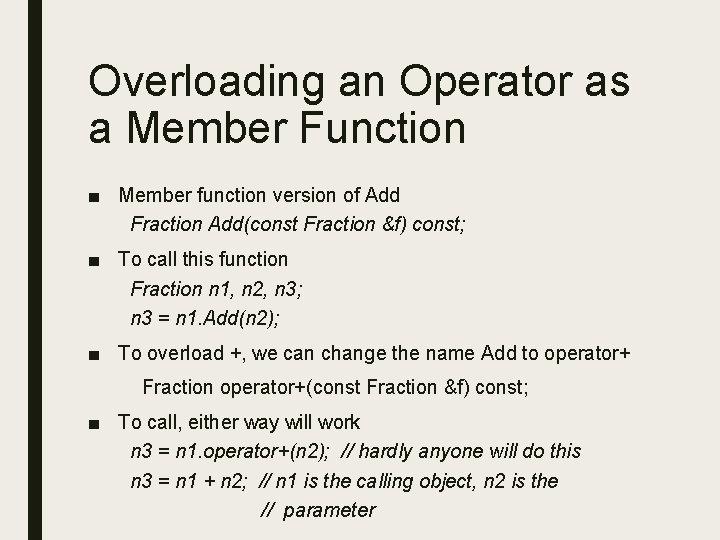Overloading an Operator as a Member Function ■ Member function version of Add Fraction Add(const Fraction &f) const; ■ To call this function Fraction n 1, n 2, n 3; n 3 = n 1. Add(n 2); ■ To overload +, we can change the name Add to operator+ Fraction operator+(const Fraction &f) const; ■ To call, either way will work n 3 = n 1. operator+(n 2); // hardly anyone will do this n 3 = n 1 + n 2; // n 1 is the calling object, n 2 is the // parameterDefinition of operator+ Fraction: : operator+(const Fraction &f) const { Fraction r; r. numerator = (numerator*f. denominator) + (f. numerator*denominator); r. denominator = (denominator*f. denominator); return r; }Example Code ■ http: //www. cs. fsu. edu/~myers/cop 3330/examples/oo/frac 2/frac. h class Fraction { public: Fraction operator+(const Fraction &f) const; … private: … };frac. cpp … Fraction: : operator+(const Fraction &f) const { Fraction r; r. numerator = (numerator*f. denominator) + (f. numerator*denominator); r. denominator = denominator*f. denominator; return r; } …main. cpp #include <iostream> #include “frac. h” using namespace std; Int main() { Fraction f 1, f 2, f 3(3, 4), f 4(6); … cout << “n Now enter first fraction: “; f 1. Input(); cout << “n. You entered “; f 1. Show(); cout << “n Now enter first fraction: “; f 2. Input(); cout << “n. You entered “; f 2. Show();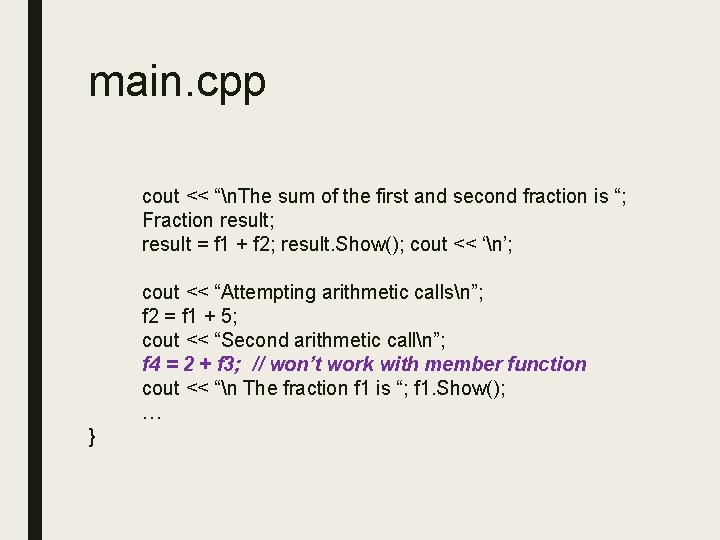main. cpp cout << “n. The sum of the first and second fraction is “; Fraction result; result = f 1 + f 2; result. Show(); cout << ‘n’; cout << “Attempting arithmetic callsn”; f 2 = f 1 + 5; cout << “Second arithmetic calln”; f 4 = 2 + f 3; // won’t work with member function cout << “n The fraction f 1 is “; f 1. Show(); … }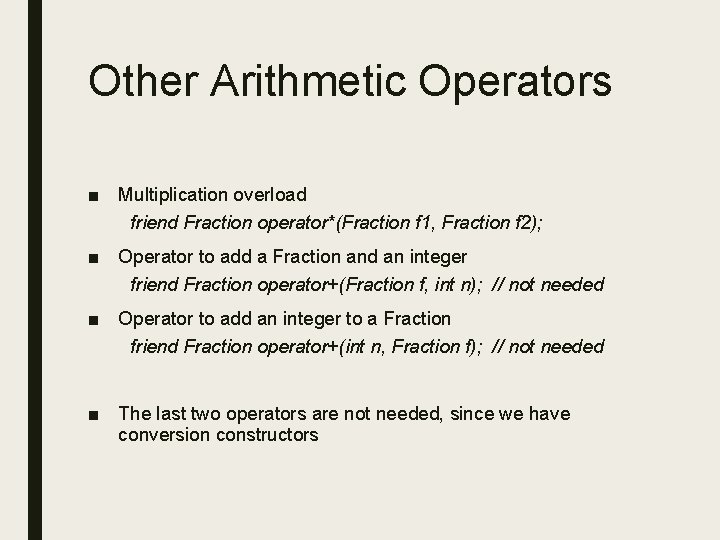Other Arithmetic Operators ■ Multiplication overload friend Fraction operator*(Fraction f 1, Fraction f 2); ■ Operator to add a Fraction and an integer friend Fraction operator+(Fraction f, int n); // not needed ■ Operator to add an integer to a Fraction friend Fraction operator+(int n, Fraction f); // not needed ■ The last two operators are not needed, since we have conversion constructors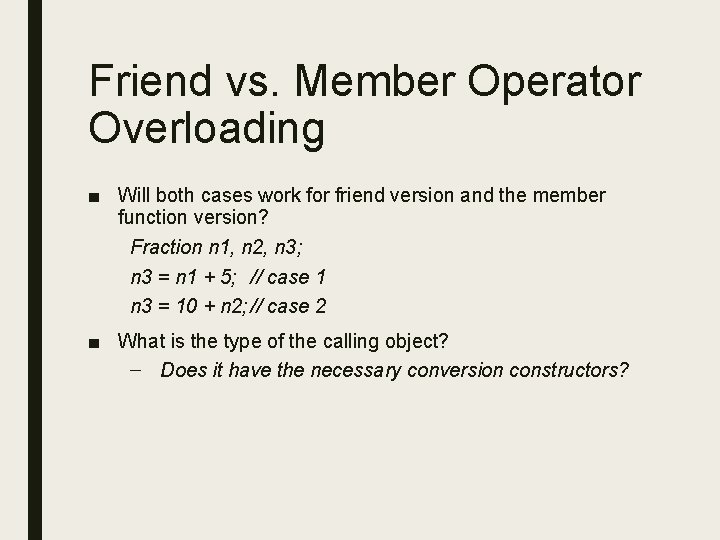Friend vs. Member Operator Overloading ■ Will both cases work for friend version and the member function version? Fraction n 1, n 2, n 3; n 3 = n 1 + 5; // case 1 n 3 = 10 + n 2; // case 2 ■ What is the type of the calling object? – Does it have the necessary conversion constructors?Overloading Comparison Operators ■ Can be overloaded either as stand-alone or member functions ■ Here is the original Equals function friend bool Equals(const Fraction &f 1, const Fraction &f 2); ■ We can write this as an operator overload friend bool operator==(const Fraction &f 1, const Fraction &f 2); ■ Here are the corresponding calls Fraction n 1, n 2; if (Equals(n 1, n 2)) cout << “n 1 and n 2 are equal”; If (n 1 == n 2) cout << “n 1 and n 2 are equal”; Hmm… Can apple == orange? Well, if we see them as fruits…Can Overload All Six ■ operator== ■ operator!= ■ operator< ■ operator> ■ operator<= ■ operator>=Overloading the Insertion << and Extraction >> Operators ■ << and >> are defined for basic types – Thus, the following code should not work Fraction f; cout << f; ■ You need to teach the computer to work user-defined typesOverloading the Insertion << and Extraction >> Operators ■ << and >> are binary operators – The first operator is always an io stream object (e. g. , cout) ■ – Thus, << cannot be defined as a member function Should be defined as outside (usually friend) functions ■ The second parameter is the user-defined type ■ Here is the overloading function friend ostream& operator<<(ostream &s, Fraction f); – Or a better version friend ostream& operator<<(ostream &s, const Fraction &f);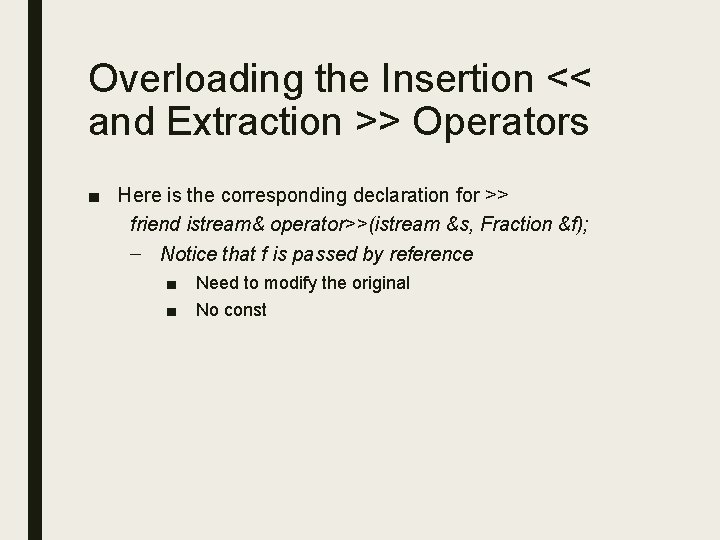Overloading the Insertion << and Extraction >> Operators ■ Here is the corresponding declaration for >> friend istream& operator>>(istream &s, Fraction &f); – Notice that f is passed by reference ■ ■ Need to modify the original No const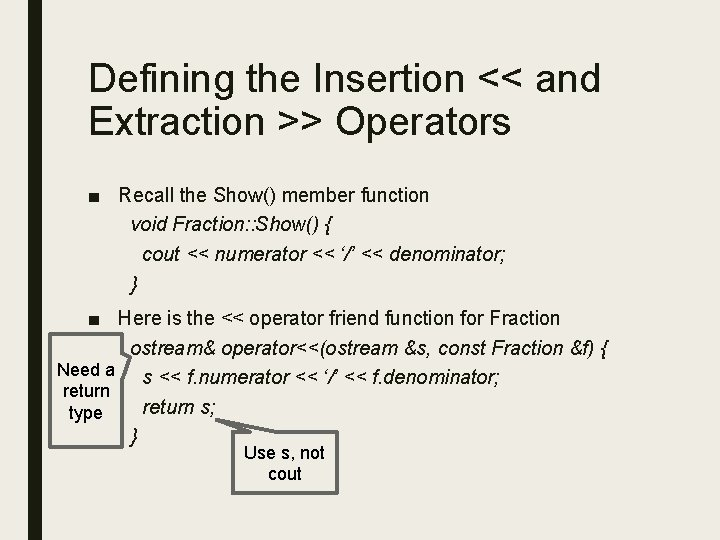Defining the Insertion << and Extraction >> Operators ■ Recall the Show() member function void Fraction: : Show() { cout << numerator << ‘/’ << denominator; } ■ Here is the << operator friend function for Fraction ostream& operator<<(ostream &s, const Fraction &f) { Need a s << f. numerator << ‘/’ << f. denominator; return s; type } Use s, not cout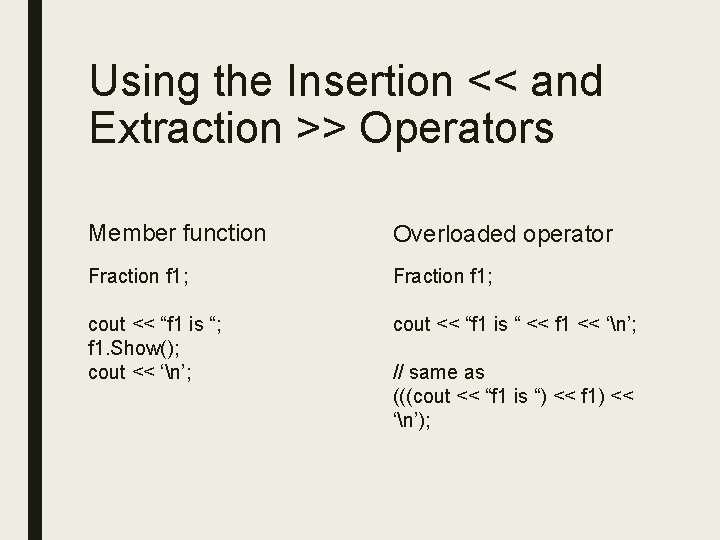Using the Insertion << and Extraction >> Operators Member function Overloaded operator Fraction f 1; cout << “f 1 is “; f 1. Show(); cout << ‘n’; cout << “f 1 is “ << f 1 << ‘n’; // same as (((cout << “f 1 is “) << f 1) << ‘n’);Example with << Overload ■ http: //www. cs. fsu. edu/~myers/cop 3330/examples/oo/frac 3/ ■ How about >> overload for Fraction? – Try it!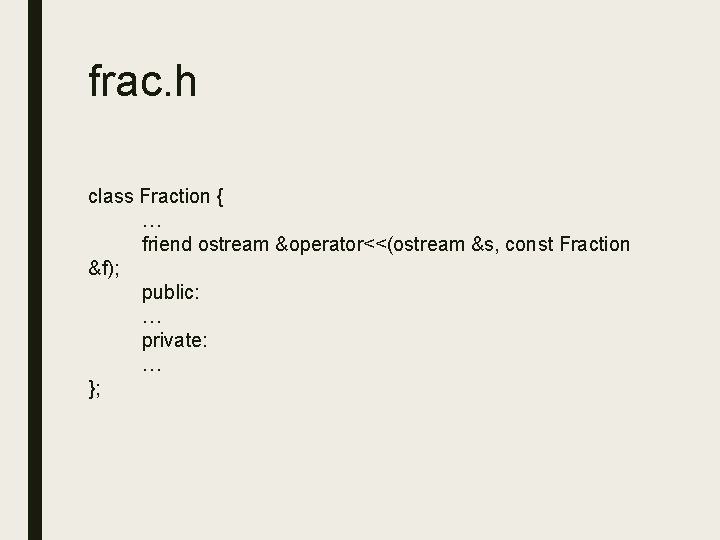frac. h class Fraction { … friend ostream &operator<<(ostream &s, const Fraction &f); public: … private: … };frac. cpp … ostream& operator<<(ostream &s, const Fraction &f) { s << f. numerator << ‘/’ << f. denominator; return s; } …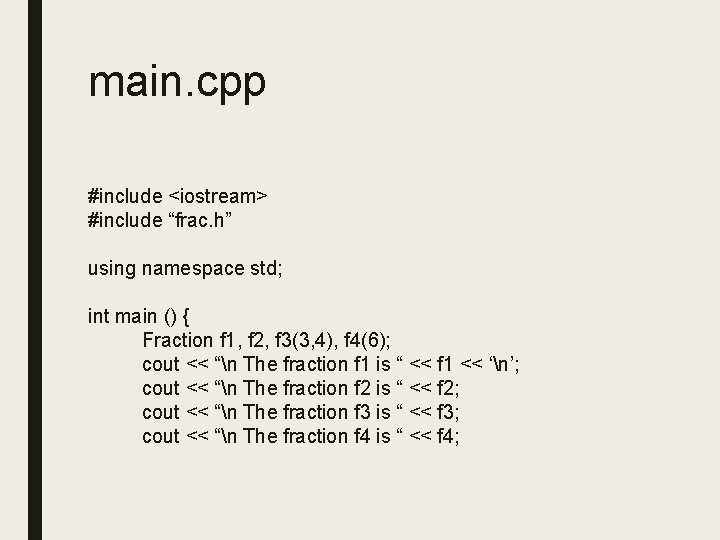main. cpp #include <iostream> #include “frac. h” using namespace std; int main () { Fraction f 1, f 2, f 3(3, 4), f 4(6); cout << “n The fraction f 1 is “ << f 1 << ‘n’; cout << “n The fraction f 2 is “ << f 2; cout << “n The fraction f 3 is “ << f 3; cout << “n The fraction f 4 is “ << f 4;main. cpp cout << “n Now enter first fraction: “; f 1. Input(); cout << “n. You entered “ << f 1; cout << “n Now enter second fraction: “; f 2. Input(); cout << “n. You entered “ << f 2; Fraction result; result = f 1 + f 2; cout << “n. The sum of the first and second fraction is “ << result << ‘n’;main. cpp cout << “n The value of fraction 1 is “ << f 1. Evaluate() << ‘n’; cout << “n The value of fraction 2 is “ << f 2. Evaluate() << ‘n’; cout << “Goodbyte!n”; return 0; }Another Example ■ http: //www. cs. fsu. edu/~myers/cop 3330/examples/complex/ – Complex numbers – i 2 = -1 ■ ■ ■ Arithmetic operators: +, -, *, / Insertion and extraction operators: <<, >> Increment and decrement: ++, --Prefix vs. Postfix Notation Prefix Postfix int j = 0; while (j < 10) { cout << ++j << endl; } // same as while (j < 10) { j = j + 1; cout << j << endl; } Int j = 0; while (j < 10) { cout << j++ << endl; } // same as while (j < 10) { cout << j << endl; j = j + 1; }Review of Complex Numbers ■ (a + bi) + (c + di) = (a + c) + (b + d)i ■ (a + bi) – (c + di) = (a – c) + (b – d)i ■ (a + bi)(c + di) = ac + adi + bci – bd = (ac – bd) + (ad + bc)i ■ (a + bi)/(c + di) = [(a + bi)/(c + di)][(c – di)/c – di)] = (ac – adi + bci + bd)/(c 2 + d 2) = (ac + bd)/(c 2 + d 2) + (bc – ad)i/(c 2 + d 2) conjugatecomplex. h #include <iostream> using namespace std; class Complex { friend Complex operator+(const Complex &, const Complex &); friend Complex operator-(const Complex &, const Complex &); friend Complex operator*(const Complex &, const Complex &); friend Complex operator/(const Complex &, const Complex &);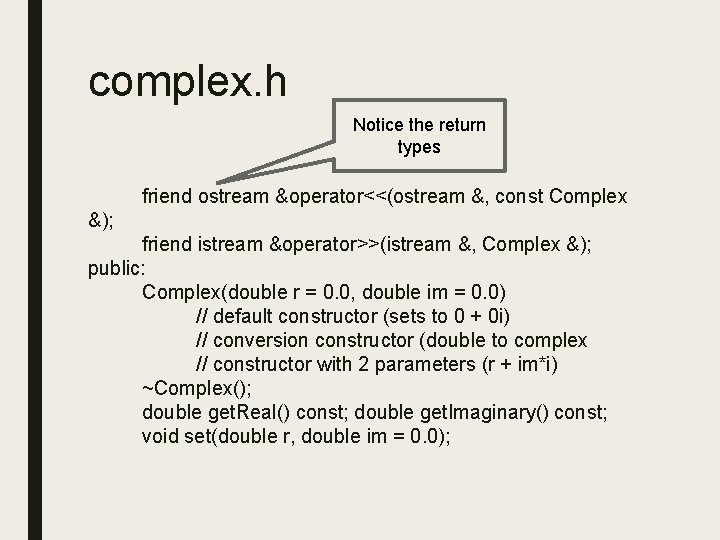complex. h Notice the return types friend ostream &operator<<(ostream &, const Complex &); friend istream &operator>>(istream &, Complex &); public: Complex(double r = 0. 0, double im = 0. 0) // default constructor (sets to 0 + 0 i) // conversion constructor (double to complex // constructor with 2 parameters (r + im*i) ~Complex(); double get. Real() const; double get. Imaginary() const; void set(double r, double im = 0. 0);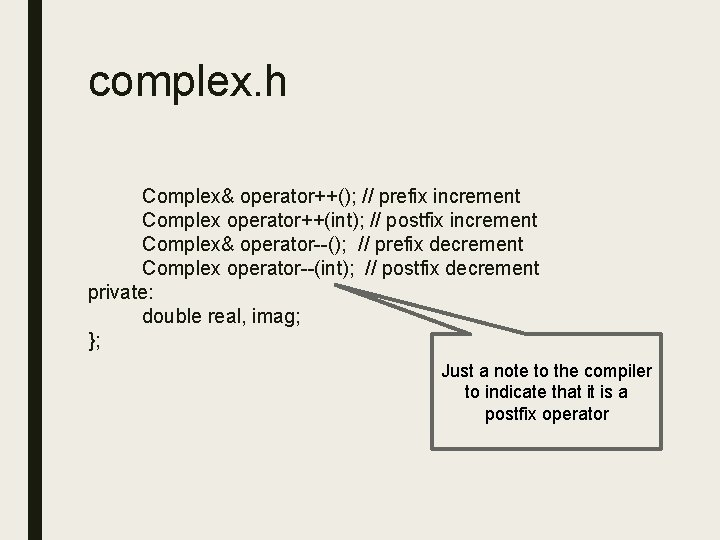complex. h Complex& operator++(); // prefix increment Complex operator++(int); // postfix increment Complex& operator--(); // prefix decrement Complex operator--(int); // postfix decrement private: double real, imag; }; Just a note to the compiler to indicate that it is a postfix operatorcomplex. cpp #include “complex. h” // iostream is already in complex. h Complex operator+(const Complex &c 1, const Complex & c 2) { return Complex(c 1. real + c 2. real, c 1. imag + c 2. imag); } Complex operator-(const Complex &c 1, const Complex &c 2) { return Complex(c 1. real – c 2. real, c 1. imag – c 2. image); }complex. cpp Complex operator*(const Complex &c 1, const Complex &c 2) { double real. Part = c 1. real*c 2. real – c 1. imag*c 2. imag; double imag. Part = c 1. imag*c 2. real + c 1. real*c 2. imag; return Complex(real. Part, imag. Part); } Complex operator/(const Complex &c 1, const Complex &c 2) { double real. Part = (c 1. real*c 2. real + c 1. imag*c 2. imag) / (c 2. real*c 2. real + c 2. imag*c 2. imag); double imag. Part = (c 1. imag*c 2. real – c 1. real*c 2. imag) / (c 2. real*c 2. real + c 2. imag*c 2. imag); return Complex(real. Part, imag. Part); }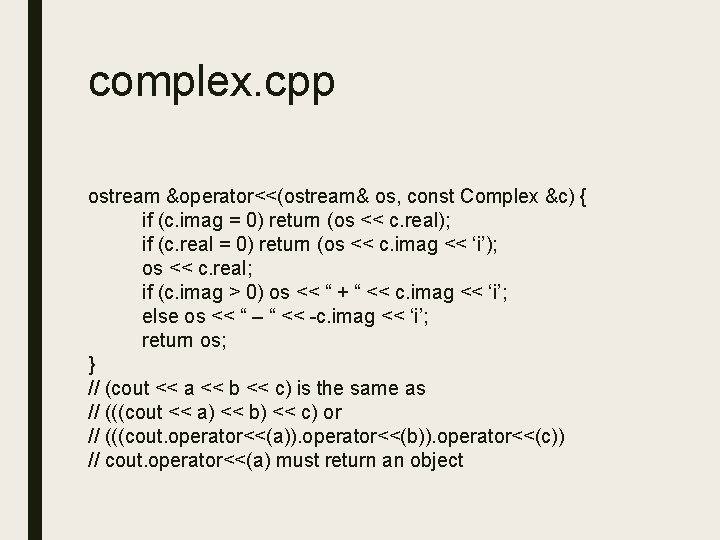complex. cpp ostream &operator<<(ostream& os, const Complex &c) { if (c. imag = 0) return (os << c. real); if (c. real = 0) return (os << c. imag << ‘i’); os << c. real; if (c. imag > 0) os << “ + “ << c. imag << ‘i’; else os << “ – “ << -c. imag << ‘i’; return os; } // (cout << a << b << c) is the same as // (((cout << a) << b) << c) or // (((cout. operator<<(a)). operator<<(b)). operator<<(c)) // cout. operator<<(a) must return an object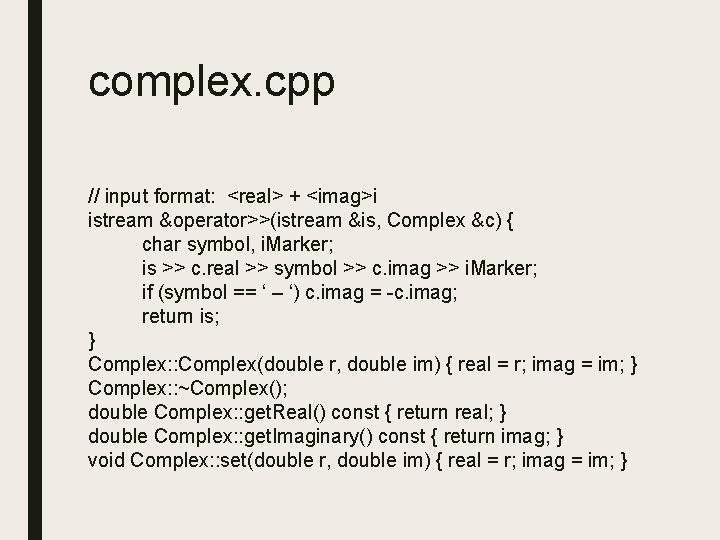complex. cpp // input format: <real> + <imag>i istream &operator>>(istream &is, Complex &c) { char symbol, i. Marker; is >> c. real >> symbol >> c. imag >> i. Marker; if (symbol == ‘ – ‘) c. imag = -c. imag; return is; } Complex: : Complex(double r, double im) { real = r; imag = im; } Complex: : ~Complex(); double Complex: : get. Real() const { return real; } double Complex: : get. Imaginary() const { return imag; } void Complex: : set(double r, double im) { real = r; imag = im; }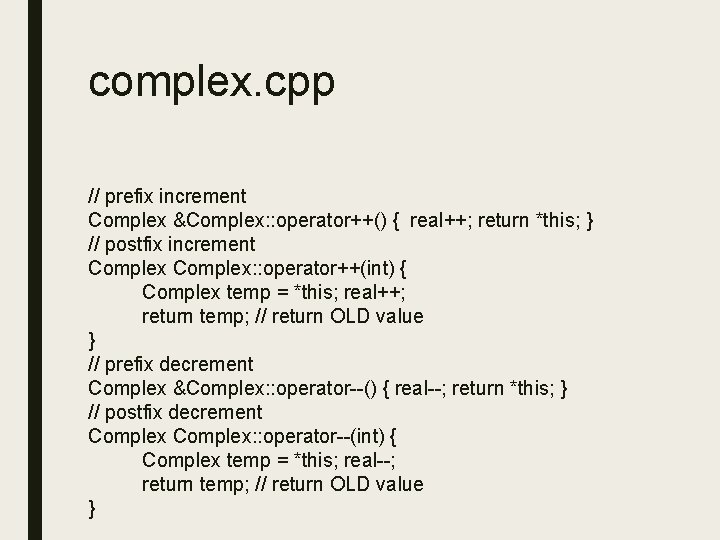complex. cpp // prefix increment Complex &Complex: : operator++() { real++; return *this; } // postfix increment Complex: : operator++(int) { Complex temp = *this; real++; return temp; // return OLD value } // prefix decrement Complex &Complex: : operator--() { real--; return *this; } // postfix decrement Complex: : operator--(int) { Complex temp = *this; real--; return temp; // return OLD value }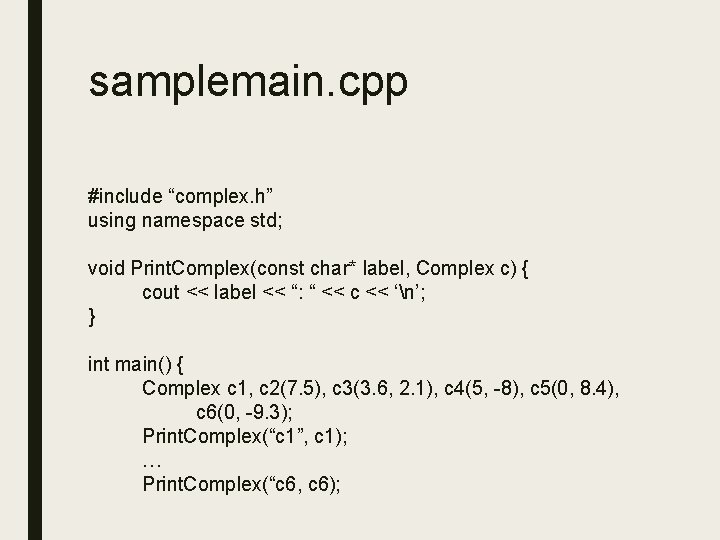samplemain. cpp #include “complex. h” using namespace std; void Print. Complex(const char* label, Complex c) { cout << label << “: “ << c << ‘n’; } int main() { Complex c 1, c 2(7. 5), c 3(3. 6, 2. 1), c 4(5, -8), c 5(0, 8. 4), c 6(0, -9. 3); Print. Complex(“c 1”, c 1); … Print. Complex(“c 6, c 6);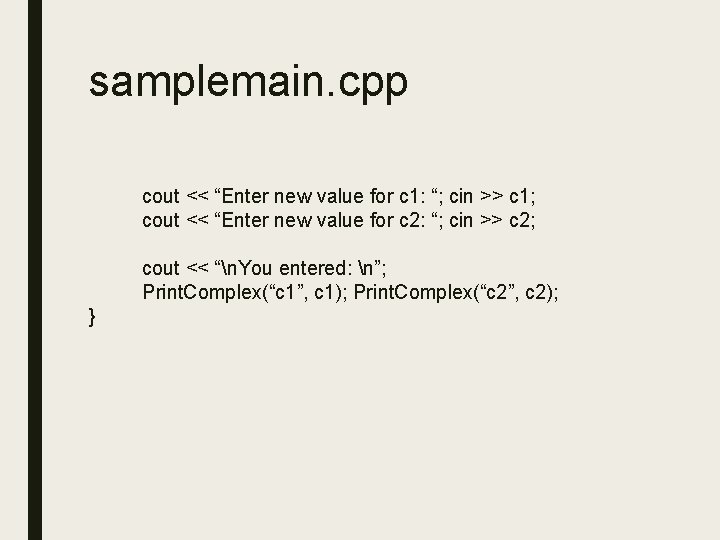samplemain. cpp cout << “Enter new value for c 1: “; cin >> c 1; cout << “Enter new value for c 2: “; cin >> c 2; cout << “n. You entered: n”; Print. Complex(“c 1”, c 1); Print. Complex(“c 2”, c 2); }